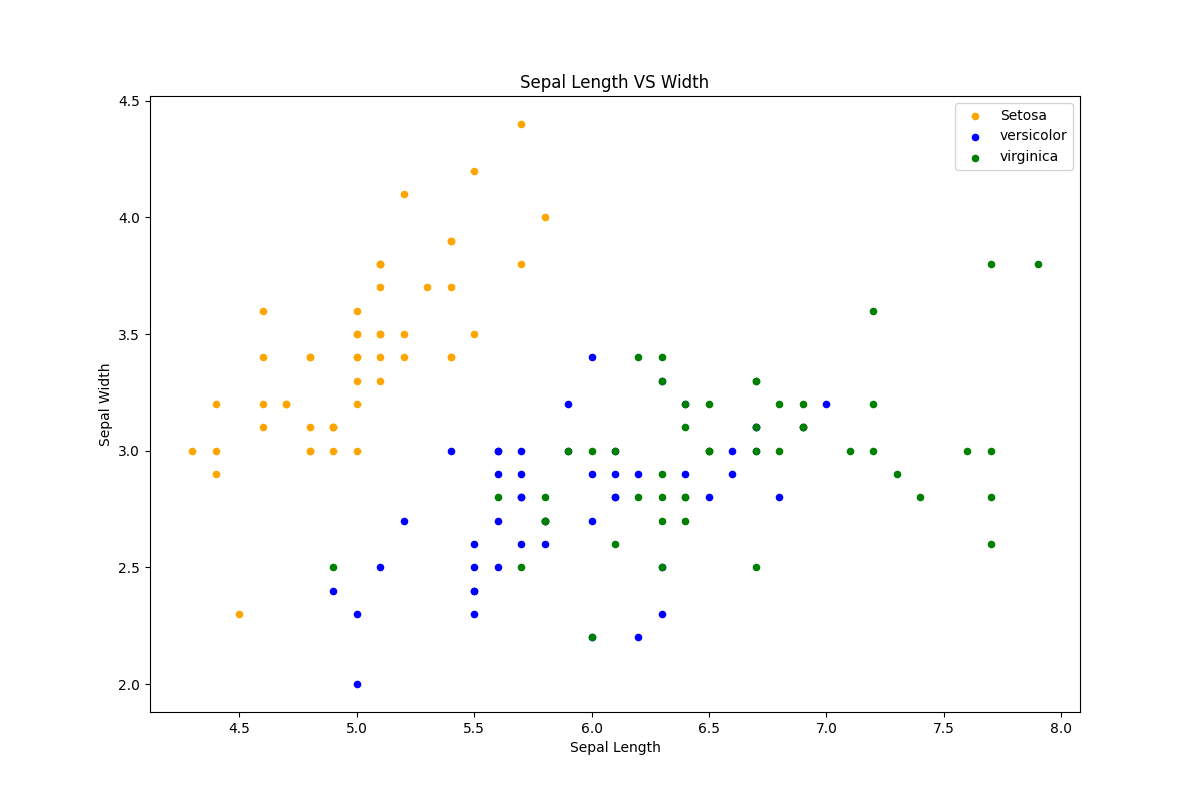﻿ Python Machine learning Scikit-learn: Create a graph to find relationship between the sepal length and width - w3resource# Python Scikit-learn: Create a graph to find relationship between the sepal length and width

## Python Machine learning Iris Visualization: Exercise-4 with Solution

Write a Python program to create a graph to find relationship between the sepal length and width.

Sample Solution:

Python Code:

``````import pandas as pd
import matplotlib.pyplot as plt
iris = pd.read_csv("iris.csv")
fig = iris[iris.Species=='Iris-setosa'].plot(kind='scatter',x='SepalLengthCm',y='SepalWidthCm',color='orange', label='Setosa')
iris[iris.Species=='Iris-versicolor'].plot(kind='scatter',x='SepalLengthCm',y='SepalWidthCm',color='blue', label='versicolor',ax=fig)
iris[iris.Species=='Iris-virginica'].plot(kind='scatter',x='SepalLengthCm',y='SepalWidthCm',color='green', label='virginica', ax=fig)
fig.set_xlabel("Sepal Length")
fig.set_ylabel("Sepal Width")
fig.set_title("Sepal Length VS Width")
fig=plt.gcf()
fig.set_size_inches(12,8)
plt.show()
```
```

Output:

``````

Python Code Editor:

Have another way to solve this solution? Contribute your code (and comments) through Disqus.

What is the difficulty level of this exercise?

﻿